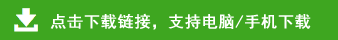# 小学四年级上册三位数乘两位数竖式计算练习题400道

三位数乘两位数竖式计算题

135×85=　  408×36=　　80×312=　　87×210=　　138×69=

82×403=　　126×89=　  203×32=　　310×25=　　336×21=

858×39=　　918×27=　　888×37=　　645×32=　　437×28=

432×46=　　966×23=　　731×79=　　980×28=　　828×36=

568×39=　　307×46=　　54×312=　　47×210=　　294×29=

689×34=　　618×88=　　372×45=　　234×46=　　613×48=